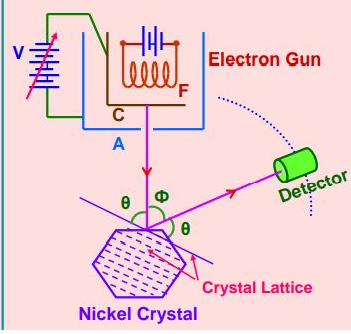# In the Davisson and Germer experiment, the velocity of electrons emitted from the electron gun can be increased by Option 1) increasing the potential difference between the anode and filament Option 2) increasing the filament current Option 3) decreasing the filament current Option 4) decreasing the potential difference between the anode and filament

S subam

As we discussed in concept

Galvanometer in Davisson Germer Experiment -

To measure the small values of current:

- whereinElectron is accelerated by applying potential difference between anode & cathode hence velocity of electron emitted from the electron gun can be increased by increasing potential difference between the anode and the filament.

Option 1)

increasing the potential difference between the anode and filament

This option is correct.

Option 2)

increasing the filament current

This option is incorrect.

Option 3)

decreasing the filament current

This option is incorrect.

Option 4)

decreasing the potential difference between the anode and filament

This option is incorrect.

Exams
Articles
Questions